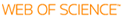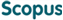### BROWSE

#### Related ResearcherJang, Bongsoo
Computational Mathematical Science Lab (CMS Lab)
Research Interests
• Numerical analysis, dynamics, fractional systems, data sciences

Efficient analytic method for solving nonlinear fractional differential equations

Cited 0 times inCited 0 times inTitle
Efficient analytic method for solving nonlinear fractional differential equations
Author
Issue Date
2014-03
Publisher
ELSEVIER SCIENCE INC
Citation
APPLIED MATHEMATICAL MODELLING, v.38, no.5-6, pp.1775 - 1787
Abstract
In this paper, we propose a new approach of the generalized differential transform method (GDTM) for solving nonlinear fractional differential equations. In GDTM, it is a key to derive a recurrence relation of generalized differential transform (GDT) associated with the solution in the given fractional equation. However, the recurrence relations of complex nonlinear functions such as exponential, logarithmic and trigonometry functions have not been derived before in GDTM. We propose new algorithms to construct the recurrence relations of complex nonlinear functions and apply the GDTM with the proposed algorithms to solve nonlinear fractional differential equations. Several illustrative examples are demonstrated to show the effectiveness of the proposed method. It is shown that the proposed technique is robust and accurate for solving fractional differential equations.
URI
https://scholarworks.unist.ac.kr/handle/201301/4070
URL
http://www.scopus.com/inward/record.url?partnerID=HzOxMe3b&scp=84894711208
DOI
10.1016/j.apm.2013.09.018
ISSN
0307-904X
Appears in Collections:
PHY_Journal Papers
Files in This Item:
There are no files associated with this item.